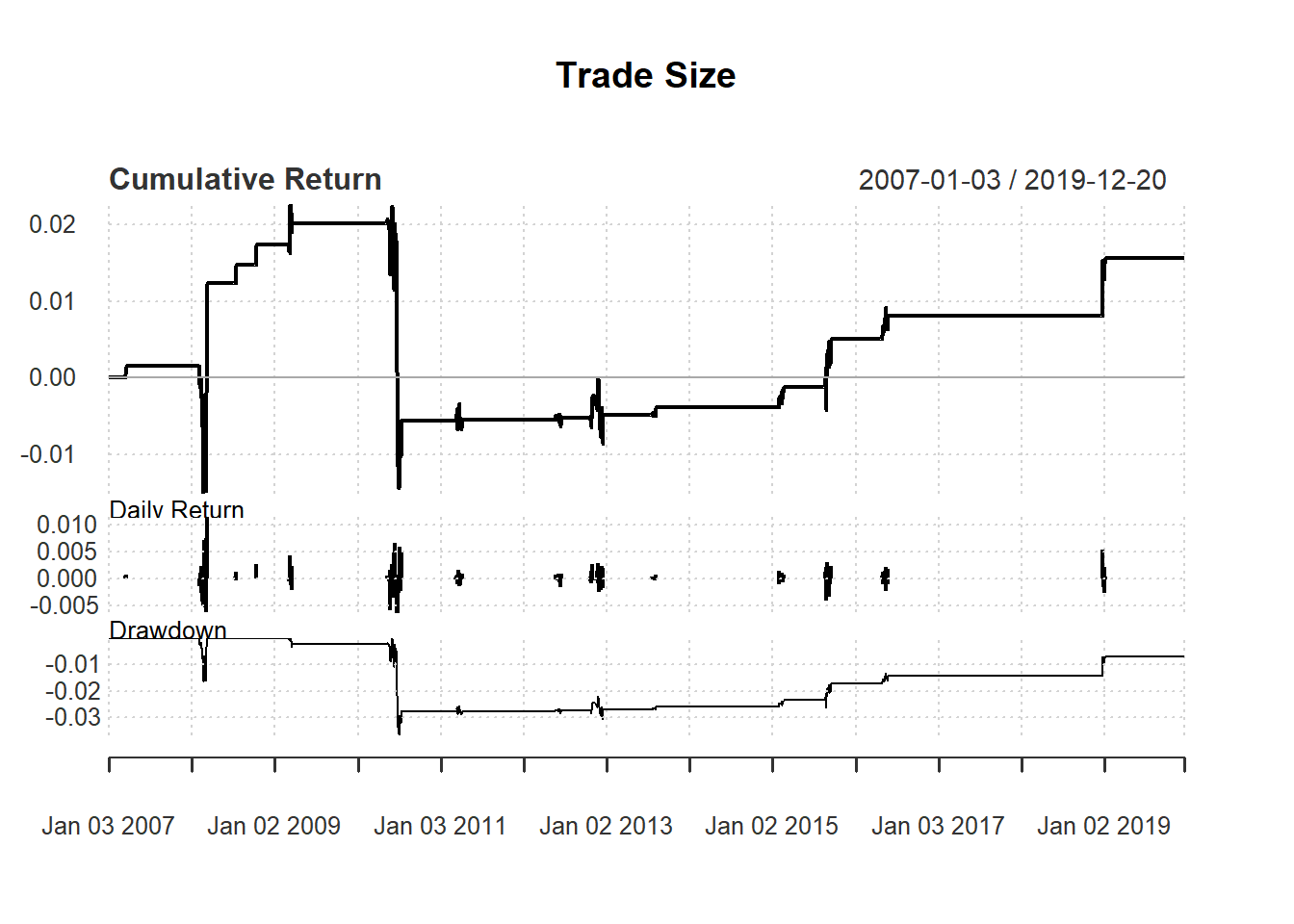Wealth: 1 million

Test the following strategy based on 14-day RSI :

• Buy one more unit if RSI <30.

• Keep buying the same if 30 < RSI < 50

• Stop trading if RSI >= 50

We will use closing price of MSFT to construct our technical indicator:

price <- Cl(MSFT)

First, we generate the techncial indicator:

day <-14
rsi <- RSI(price, day)

Second, we generate trading signal together with size:

signal <- c()
signal[1:(day+1)] <- 0

for (i in (day+1): length(price)){
if (rsi[i] < 30){  #buy one more unit
signal[i] <- signal[i-1]+1
} else if (rsi[i] < 50){  #no change
signal[i] <- signal[i-1]
} else {         #sell all
signal[i] <- 0
}
}
signal<-reclass(signal,price)

To take trade size into account, we need to keep track of wealth:

qty <-1000 #trading size

wealth <-c()
wealth[1:(day+1)] <- 1000000

profit <-c()
profit[1:(day+1)] <- 0

return<-c()
return[1:(day+1)] <- 0

Here, wealth is the market value of the portfolio, profit is the absolute amount of profit each day, and return is the daily percentage profit.

Close <- Cl(MSFT)
Open <- Op(MSFT)
ret<-reclass(return,price)
charts.PerformanceSummary(ret, main="Trade Size")